## Introduction

The financial crisis has made clear that we need empirical measures of systemic risk that are able to capture some precursors of systemic events such as the building-up of financial instability1,2,3. At the same time, it has been increasingly recognized that financial networks play an important role for (in)stability properties of the financial and economic system4,5,6,7,8,9,10. One of the networks that has been recently investigated in terms of its relations to systemic risk is the network of derivative contracts11,12,13, in particular the network of Credit Default Swaps contracts14,15. Because network data regarding derivative contracts including CDS are typically confidential and hardly accessible even to regulators, there has been a growing interest in networks constructed from time series of financial indicators building on a stream of works that has already a long tradition16,17,18,19,20,21,22,23. Therefore, an important question concerns to what extent these networks are suitable candidates to provide measures of the building up of instabilities in financial markets.

In this paper, unlike other works that look at equity returns or equity volatility, we start from the daily time series of Credit Default Swaps (CDS) spreads that are considered to reflect more directly the default probability of the related institutions. In particular, our dataset encompasses a total of 176 top firms in the financial sector in the period from 2002 to 2011. The complete table of institutions names and tickers is reported in the Supplementary Information. To broaden the robustness of the results, we compare 5 different methods among the most commonly used in the literature to construct networks from time series (e.g., linear and non-linear correlation, Granger causality and ε-draw-up).

We study the evolution of these networks over time and we observe a structural change at the very onset of the crisis, end of 2008, but not before (the “crisis” period is defined using the independent and standard definition of “recession” i.e. two consecutive quarters of negative GDP growth). The financial networks have in general high density (i.e. the ratio of the links present over the possible ones) as they are built from cross-correlation of pairs of financial time series. Such correlation levels are most of the time statistically significant17,18,19,20,21,22,23. At the onset of the crisis the networks become even more dense, have stronger links and their minimum spanning trees display shorter average distance. We then take the networks as a proxy of the possible interdependencies among the institutions over which the CDS are issued and we carry out an exercise of simulated stress-tests. We apply the computation of Group DebtRank to the networks in the case of a small shock hitting all the institutions. Group DebtRank is a measure introduced in a previous work5 that estimates the impact on the whole system due to a shock hitting several nodes of a financial network. It is a measure of the potential systemic loss, conditional to a given shock. Therefore, the higher the Group DebtRank the higher is the systemic risk meant as probability of a systemic event (see Methods). Similarly to the topological analysis, we observe a clear increase of Group DebtRank from the end of 2008, but not before. We finally couple the shock used in Group DebtRank to a macroeconomic indicator reflecting the potential loss of financial products depending on house prices in the US and In this case we find a building up of the values of Group DebtRank during the several years before 2008.

Overall, the results suggest that not only the raw time series of CDS spreads did not anticipate the crisis but also networks constructed from CDS daily time series are not able to capture, alone, the building up of systemic risk in the financial system. We do not investigate here why this is the case, although these results raise a number of interesting questions regarding the functioning of the CDS market and the trading of risk within the financial system. The results also suggest that incorporating appropriate macroeconomic indicators in the network measures could be a promising way to detect instabilities.

## Results

### Evolution of network topology

By looking at the daily time series of Credit Default Swaps (CDS) spread in Figure 1 (left), we observe that the period 2002–2007 is characterized by low values of the spreads and low volatility (with some minor burst in 2005), while from the beginning of 2007 spreads rapidly increase reaching values sometimes 10 or more times larger than they were before. The same figure shows also the behaviour of the average market capitalization for the same set of companies. After a steady increase in the period 2002–2006, from the beginning of 2007 the average value decreases steadily and by the end of 2008 drops by almost 50%. The point of inversion for the two curves occurs almost at the same time. For the sake of our successive analyses, we compare, in Figure 1 (right), the ratio of the house price index over the household disposable income, denoted as HPI. The period under examination is about 30 years, from 1983 to 2011. Roughly speaking, this ratio provides an estimate for the average price of a house in terms of the average income available to households to purchase a house (see more details in Methods). The beginning of 2007 is also the moment when the HPI over income ratio reaches a peak of about 14 and starts to decrease after 15 years of upward trend. The average value of the ratio over the 30 years period is 12. This latter indicator will become relevant in the final part of the analysis.

We analyse the daily time series of CDS spreads using a rolling window of 6 or 12 months (only for the Drawups/comovements method) that moves in steps of 1 week. We represent institutions associated with the CDS spreads with the nodes of a network. For each week, we then assign a link between a pair of institutions if the comovements of the two time series during the preceding 6 (12) months fulfill certain criteria (see Methods).

We repeat this procedure using six different methods among the most commonly used in the literature to construct networks from time series (i.e., linear (Pearson) correlation, correlation slope, non-linear correlation, time-lagged Pearson correlation, Granger causality and drawups, see Methods).

First, we study the evolution over time of the measure (number of vertices), the density and the basic structure of the networks as measured by the average distance.

### Network measures

The size N (number of nodes) of the network is defined as the number of institutions that have at least one link to some other institution, i.e. by construction there are no disconnected nodes. This means that the time series of an institution has to comove at a statistically significant level, according to the selected network construction method, with the time series of at least another institution in order to be counted in the network. Because the definition of comovement varies across the methods, the various measures may vary as well. Finally, by construction when we discuss about a given year/period we are referring to the backward looking intervals e.g. 2007 is the period from 2006 to 2007. As shown in Figure 2 (top left), with methods (1–4) the network size increases steadily from values close to 10 in 2002 up to about 130 in 2010, with a plateau in 2005.

The plot of the link density shows an increase in the measure during the period 2007/2011 less evident for Drawups and lagged Pearson (that shows an almost constant link density during the years). The average spanning tree path length is similar for all methods except for the lagged Pearson that does not reproduces correctly even the size of the network. This is probably due to the lack of significant correlation between the curves after a few days i.e. the lag destroys the correlation and makes the links weaker.

Notice that the links in the network are directed in the cases of time-lagged Pearson, Granger causality and Drawups methods, while they are undirected in the three other cases.

### Density

The density of the networks is simply the fraction of links that exists out of the number of possible links. As shown in Figure 2 (bottom left), the networks have in general high link density, from 20% to 50% depending on the network construction method and on the time period. Notice that we consider only the links that are statistically significant (see Methods). At the onset of the crisis the density increases. This implies that the number and the intensity of the interdependences among nodes in the network increase; a feature that is relevant for distress propagation, as we will see later on.

### Average degree and average distance

Because many of the networks are very dense, in line with previous works on correlation networks17,18, we extract the minimum spanning tree (MST) of each undirected network. The average path among nodes in the MST is a measure of the distance among nodes in the network along a set of strong links. As shown in Figure 2 (bottom right), we observe a sharp increase of this quantity in the MST in 2003; after that it increases only slightly.

Although the statistical test to retain the links is the same, we notice substantial differences among the different methods when we look both at the average degree and the density. Notice that the average degree equals N-1 times the density, where the network size N varies in time.

The average degree (Figure 2, top right) is close to 10 in 2002, reaches a first peak around 2005, drops slightly in 2006, increases again until the first half of 2010 up to values twice as large as in 2005 and finally drops at the end of 2010. In general the directed networks (lagged Pearson, Granger, drawups) yields sparser networks. The networks obtained with the Granger causality method show some cyclic behavior over time in size and density. On average, we observe a gradual growth over time of the number of nodes, the number of links and their weights. We also observe a structural change in the period 2005–2007 in the sense that the average degree and the density drop down. Notice that according to the rolling window method, the interval is always backward-looking, e.g. 2007 means August 2006 to July 2007. This finding is relatively robust across methods.

### Group DebtRank analysis

In Figure 3 (left) we report the values of the CDS spreads over time in semi-log scale. We observe that the minimum in the spread is reached in the beginning of 2007 followed by a sharp and steady increase. In linear scale, the values in the period 2002–2007 would be almost invisible compared to those afterwards.

As a second step of the analysis, we consider the links in the networks (originated as described in the previous section) as a proxy of the interdependencies among institutions and we carry out an exercise of simulated stress-tests. It is worthwhile noticing that cross-border exposures between top financial institutions in the world are undisclosed even to regulators. Furthermore also within-border exposures are only partially known to regulators in certain countries. Therefore, proxies inferred from time series are a necessary instrument to investigate systemic risk.

We apply the computation of Group DebtRank to the networks constructed as in the previous section in the case of a small shock hitting all the institutions. Group DebtRank is a measure introduced in a previous work24 that, assuming to know mutual exposures and levels of capital across banks, computes the impact of a shock hitting several banks taking into account the network effects (see Methods). It measures the potential systemic loss, conditional to a given shock. Therefore, ceteris paribus, the higher the Group DebtRank, the higher the systemic risk (meant as probability of a systemic event, as shown in Methods).

Since the matrices of exposures among institutions over time are not available, we need to analyse different scenarios. We are not aware of a consistent way to make the strength of the links obtained with the various methods comparable among each other. Actually, this is unsolved problem in the field. Here, we take the strength of the links computed with the various methods and we rescale them all by a parameter α in order to have them varying in a comparable range. Therefore, the plots in Figure 3 (right) may use different values of α depending on the method. The purpose of this plot is to illustrate the behaviour over time across methods. While the spreads are a measure of individual default risk of institutions, Group DebtRank measures the systemic impact of a shock on all of them taking into account the network effects. It is therefore interesting to compare the values of Group DebtRank (Figure 3, right) with the values of the spreads from which they are derived (Figure 3 left). In both cases the years 2005–2007 correspond the minimum of risk.

Despite the differences in the absolute values of Group DebtRank, some findings are common to the six methods. First, relative to the values of Group DebtRank in the crisis period of 2008–2011, the values of Group DebtRank are substantially lower than in the years before. Second, all methods yield also a peak of Group DebtRank in 2004–2005. With Granger causality and time-lagged Pearson correlation, such a peak is about 50% smaller than the values reached after 2008. Third, all methods show a clear drop of Group DebtRank in the years 2005–2007. Remarkably, this means that, regardless of the method used to construct the network, the systemic risk estimated from the network of CDS comovements was at a minimum right before the onset of the crisis. In summary, we observe a clear increase of Group Debt Rank from the end of 2007, while before 2007 the network constructed from the signal of the CDS market do not indicate any building up of risk.

### Group DebtRank and house price index

As a last exercise, we want to modulate the shock entering in the Group DebtRank computation with some macroeconomic indicator. Many practitioners agree that the asset class related to the housing sector is often a good predictor of bubbles and crises. In particular, it was responsible for triggering the credit crisis in 20083 since most financial institutions had increasing amounts of mortgage-derived assets in their balance sheets in the period before 2008. When they then tried to sell them, the price plummeted and eventually many of those institutions had to rely on rescue emergency programs5.

The scenario we have in mind is the following. Assume that the financial institutions in the data set have a certain exposure to such class of assets in their balance sheets and assume that, if the bubble bursts on day t, then prices drop rapidly down to a “baseline” value. We then ask the question: What would be the impact of such a shock to the system taking into account the network effects? (with the caveats mentioned above). Then we vary the day t at which the shock occurs across the whole time range of the data set. In the exercise, we take the ratio of the house price index (HPI, corrected for inflation) over household disposable income index, normalized in such a way that the long term average is the baseline for the 100% value (see Methods). The aim is to have a rough estimate of the maximum potential loss in value of the financial assets that depended on house prices in the US, such as mortgage-backed securities. Using a common practice in economics in which an index is compared to its long-term average baseline, we take as the latter the average value that prices had in the previous period.

It is true that prices did not drop in the space of weeks when the bubble burst (it actually took 3 years). However, what is similar to the scenario depicted above is that as prices started to decrease they continued to do so and eventually dropped down to roughly the mentioned baseline value by the beginning of 2010. The moment at which prices start to decrease would have been of course impossible to forecast. However, it seems reasonable to say that when the bubble bursts prices tend to fall down to the baseline value, although it would be impossible to make precise statements on this. In this paper, these considerations are simply a motivation to model the size of the shocks hitting the system. Essentially, we are coupling the network effects with a macroeconomic indicator reflecting housing prices.

In Figure 4, the solid lines represent the values of Group DebtRank, i.e. the systemic impact, obtained combining network effects and macro shock. The dashed lines refer to the systemic impact of the macroeconomic shock alone, without the network effect. Red lines refer to a scenario with a stronger shock (i.e. 20% of losses for each institution modulated by the external HPI levels, see Methods), while blue lines refer to a smaller shock (10% of losses for each node). Higher values of Group DebtRank stand for higher systemic risk. The baseline of the external shock (here the dashed lines) is a curve that shows an increase in the house price levels from a value of about 12 (2002) to 14 (beginning 2007), i.e. a rise of about 15% and finally a dramatic drop of about 30% to the end of 2011, see for reference Fig. 1 (Right). In this plot the long-term average of that curve (11.9) is used as reference value (see also methods).

Figure 4 (left) shows the results we obtain with the networks constructed using the Pearson correlation. As in Figure 3 (right), there is a build-up and a precursory peak in 2005, a tranquil moment in 2006 and a sharp increase in 2007–2009. Figure 4 (right) refers to the network constructed with the drawup method, where we also observe a build-up of the values of Group DebtRank already several years before 2008. There is still a peak in 2005 and a minimum in 2006 but the increase in 2007–2009 is less sharp and follows an upward trend on a longer period. In both cases the network effect can be very strong, as it amplifies the effect of the macro shock by a factor of about 4 as in the scenario depicted in the figure. The build-up observed in Figure 4 can be explained in the following way: the deviation over time of the asset prices from the baseline keeps increasing from about 1999 until about 2008, translating into an increasing size of the shock that, in our exercise, hits the system at any time t. On the one hand, the house price index decreases after 2008 and thus the size of the shock decreases accordingly. On the other hand, from 2007 the number of links increases (see Figure 2 bottom right) as well as their weights (plot not shown). As a result of the combination of these two trends, the values of Group DebtRank keep increasing after 2007 because the network effects are stronger than the decrease in shock size (see the dashed lines in figure 4 corresponding to the case of no network effect).

## Conclusion

Overall, our results suggest that networks constructed solely from CDS time series are not able to capture the building up of systemic risk in the financial system. This is at odd with the idea that the CDS market should be pricing the risk of distress of the institutions for which CDS are traded. The fact that CDS spreads themselves did not show any early warning had attracted already some attention. Some authors suggest that the increase of house prices all over the US was anomalous and that there was no consensus on the consequences that the burst of the housing bubble could have2. In particular, there was confidence that the new financial instruments, including the CDS themselves, could mitigate the risk25. In principle, the networks of CDS comovements could have carried additional information, which was not directly visible from the time series. Our findings indicate that this is not the case. We do not investigate here the reasons of this state of affairs, we only suggest that it may deserve further attention.

Moreover, our findings show that reconstructing networks from time series poses a number of challenges. There are several methods in the literature. It is not clear how (and maybe it is not even possible) to compare the values of the link weights across the various methods. Therefore, it is not clear how to compare the results of a same stress test conducted on networks obtained with different methods. This is a fundamental challenge if we aim at increasing the robustness of our assessments of systemic risk starting from time series data.

We observe that the three methods yielding undirected networks (Pearson, Pearson slope, non-linear correlation) give systemic impact curves that are closer to each other than to those obtained with the three methods yielding directed networks (time-lagged Pearson, Granger causality and drawups). However, the results we find are not always coherent across methods.

This last finding contributes some constructive criticism to a stream of recent works on the systemic importance of financial institutions, which use networks constructed using a single method – typically undirected networks obtained with Pearson correlation, or Granger causality30 and apply measures not specifically designed to capture financial risk (e.g. eigenvector centrality).

Furthermore, our results also show that incorporating appropriate macroeconomic indicators in the network measures could be a promising way to detect the building-up of systemic instabilities in the financial system as demonstrated by our exercise. Notice that network effects in the financial system can amplify the impact of a macro-shock by several times. Thus, basing the computations on the macroeconomic indicator alone would yield a substantial underestimation of systemic risk.

We also observe a large variance on the estimated systemic risk depending on the severity of the scenario considered. This means that when fundamental information on exposures is undisclosed, regulators are not in the position to make reliable estimates of systemic risk based on network data reconstructed from time series. The issue of how much data disclosure is necessary to improve this situation is going to be a challenging question for future research on systemic risk.

## Methods

### Datasets: HPI and CDS

The data of the house price index (HPI) are freely downloadable from the federal housing finance agency (http://www.fhfa.gov). HPI is defined as the ratio of the house price index over the household disposable income26. This captures by how many times the average cost of a house exceeds the yearly average income of a household. For more details see the reference above.

The dataset we used for the CDS consists of 176 daily time series of CDS spreads associated with top financial institutions in US and Europe with a maturity tenure of 5 years. The complete table of institutions names and tickers is reported in the Supplementary Information. The set refers to single entity CDS, denominated in euros and in dollars, curves are in basis points. Each time series ranges from 2nd January 2002 to 30th November 2011.

Missing data points and non-trading days are treated as follows.

1. 1

we compute the fraction of non missing points in each segment of the rolling window. If in the current window this fraction is larger than 30%, the time series is not considered and the cross-correlation of this time series with all the other series is set to 0.

2. 2

if instead in a given window two series have less than 30% missing points then the correlation between them is computed but using only those days when both CDS are simultaneously traded.

We represent institutions associated with the CDS spreads with the nodes of a network. For each week, we then assign a link between a pair of institutions if the comovements/correlation of the two time series during the previous 6 months fulfil certain criteria described further below.

Because for each week, we need to be able to look into 6 or 12 months back in the past, our results start from 1st July 2002 or 1st Jan 2003 (Drawups method). We have noticed that, from the end of 2010, several CDS are not traded anymore or data points are missing in the dataset. To ensure that a time series is suitable to be used in the computation, we require that each window (182 days) has at least 30% of non-null data points, that is 54 days with non null data points. The final part of the time series does not fulfil this requirement. Therefore, the computation ends on 16th May 2011. Overall, we construct about 450 weekly networks. We repeat the construction procedure using five different methods for measuring the comovements, as described in the following. The only exception to the use of the log-returns is the case of the method based on the ε-drawups where the co-movements are extracted from the original spread time series. The same method is also the only case in which we use a window of 12 months instead of 6 months.

The original daily time series are transformed into daily log-returns, as commonly done in the finance literature, according to the formula. The use of the log-returns decreases the variance of the sample and makes easy the randomization of the sample, used to build the confidence level tests.

### Network construction method based on Pearson correlation

There is a body of works describing the construction of networks from time series by means of some variant of the basic definition of Pearson correlation17,18,20,27: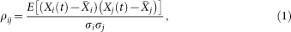where Xi(t) and Xj(t) are two vectors of time series data points (e.g., [Xi(t1), Xi(t2), …, Xi(tN)]) and σi and σj are the respective standard deviations. A link is assigned between the nodes i and j associated to the two time series if they show a significant value, ρij, of correlation (positive or negative). As we are using finite size time series (6 months = 182 days) of at least 30% of 182 = 54 non null points, we need to ensure that the correlation level is significant for samples of size 54. For larger samples the acceptance level of the correlation is lower as the small sample effect tends to disappear. This limit is then the most conservative way to filter out the non spurious correlation of the small samples, larger samples will be non randomly correlated at that level. We create a simple statistical test to calculate the 95th quantile level of the ρ distribution of samples (obtained randomly from the original, actual population of log-return prices) of size 54. With 10000 samples of length 54, the critical level of ρ at the 95th quantile is 0.22. For this reason the correlation between two time series to be significant must be larger than 0.22 (or below −0.22 for the negative value). As the value of ρij in Equation (1) ranges in the interval (−1, 1), it is useful to remap it into the interval (0, 1) following17 (with a slight modification):The quantity wij is the weight of the link, which increases with the correlation.

### Network construction method based on correlation

slope A variation of the previous method consists in performing a linear fit between the two time series under examination. If the correlation is significant, the slope of the fitted line can be positive or negative depending from the orientation of the line. Since the slope is not limited to a finite interval, a cutoff must be selected according to the distribution of the slopes: the interval (0, 2) is large enough to accommodate more than the 95% of points (see SI for the graph). Finally we rescaled linearly the weights from (0, 2) to (0, 1). In order to build the network we use the eq.(2) after having filtered out the non significant correlation levels.

### Network construction method based on time-lagged Pearson correlation

Network construction method based on time-lagged Pearson correlation. The time-lagged Pearson correlation method consists in computing the ordinary Pearson correlation as described above but with the two time series shifted by a variable time interval (from 1 to 5 days). The correlation ρ(Xi(t), Xj(t))Δt is estimated between series Xi(t) and Xj(t + Δt) where 1 < Δt ≤ 5 and averaged across the five values of Δt. This value is then assigned as weight wij of the link between node i and j.

Network construction method based on Brownian correlation Another method we use is based on a non-linear correlation measure known as Brownian correlation, or distance correlation28. The Brownian correlation captures non linear contributions especially for the larger movements. The most important property of this measure is that a value of zero distance correlation implies that the two random variables are independent, in contrast with the Pearson correlation where with a value of zero the two random variables could be still dependent. The mathematical formulation of this measure starts with the definition of the distance covariance. Let Xa(t), Xb(t) be two time series.

We compute the Euclidean distances between all possible pairs of data points in the series: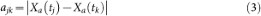We now define the matrices A, B whose elements are: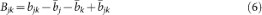where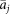is the average on the j-th row of aij,is its average on the k-th column and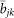the average on the whole matrix. The distance covariance is then defined as: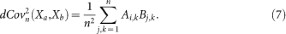The definition of the distance correlation is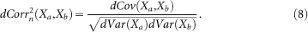The interesting property of this definition is that dCorr is bounded in the interval (0, 1) and it is higher when the dependence between Xa and Xb is stronger. We filter the dCorr with the same finite size test of the Pearson correlation. Using a significance level of 95% we obtain 0.4 as critical value for dCorr.

### Network construction method based on Granger Causality

Another approach is to use the Granger causality. We fit the time series Xi(t) with a vector whose elements are defined as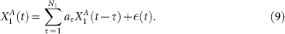We ask if the time series Xi(t) can be “caused” by the time series Xj(t). To this end, let us consider also for the series Xj(t) its fit through autoregression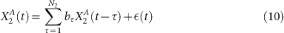where the N1 coefficients aτ are determined to fit Xi(t) and the N2 coefficients bτ are determined to fit Xj(t). The Granger causality measures the improvement in the error estimation (measured as a variance gain) obtained fitting the series Xi(t) with a new autoregression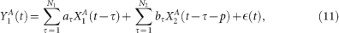where p measures a time delay. If the new fitting series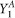has a lower error (smaller variance) than the one of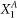, then we say that Xj(t) Granger-causes Xi(t).

In order to measure the extent of the variance reduction, we use the classical coefficient of determination defined as: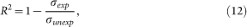where σexp is the “explained variance” (i.e. the variance of the joint model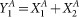), while σunexp is the variance of. If there is some variance reduction with the joint model, i.e. 0 < σexp < σunexp, then R2 (0, 1), with higher values of R2 implying higher likelihood of Xj(t) Granger-causing Xi(t).

In our analysis, when we refer to networks constructed using the method based on Granger-causality, we assign as weight wij of the link between institution i and j the coefficient of determination R2 computed between the time series of two CDS spreads for institutions i and j.

### Network construction method based on drawups

Finally, we also use a modification of the ε-drawup method23. This method allows to establish if two series are co-moving during the same time window in a statistically significant way.

Differently from the methods described above, we do not start from the time series of log-arithmic returns but from the original spread time series. An ε-drawup is defined as an upward movement (i.e. local minimum, local maximum and subsequent local minimum) in which the amplitude of the rebound (i.e, the difference between the local maximum and 2nd local minimum) is larger than a threshold ε. This quantity is tuned as a function of the variance in a window of τ days preceding the drawup. In this paper, in order to avoid the necessity to choose a value for ε, we introduce a peak detector.

We first verify that in the time series the autocorrelation of the returns of the spread become negligible already after 5 days (i.e. one working week). This means that local maxima that are away from each other by more than 5 days are not due to autocorrelation. In order to select such maxima we apply a peak filtering algorithm that smoothes out the local maxima within the 5 days interval and identifies the most prominent peaks in a window of 5 days. The peak detector is a standard set of methods used in the Python library mlpy based on the work29 to find the extrema within a rolling window of length w. The code is conceived to avoid the initial selection of the starting point, as it selects for the starting point of the rolling window (w = 5) the initial, most prominent, peak.

The drawups in the series i must be compared to the drawups of the series j. If a drawups occurs at time t in series i and another one is occurring in series j at time t + dt, where dt < τ = 5 days, we say that at that moment i and j are co-moving. Two time series may have many co-movements, but these could be an effect of a random fluctuations.

Therefore, we perform a permutation test to compute the probability of n random co-movements between the two series. We say that the number of co-movements is significant if it exceeds the number of those occurring in the random case (with 95% of confidence). Dividing the number of co-movements with the maximum number of weeks in the series (there is at most one drawup per week after the peak filtering) we obtain a number in (0, 1), which we assign as weight to the link between i and j.

### Group DebtRank

On the networks we applied the Group DebtRank methodology, a variation of the DebtRank algorithm that allows to estimate a the systemic impact of a shock to a group of nodes in the network. In particular, we focus on small shocks hitting the whole network and we want to measure the final effect, due to the reverberations through the network. We define the impact of node i on node j as α Wij, where Wij is the weight of the link assigned using the various construction methods described earlier and α is a parameter. Notice that the matrix W is, in general, neither column-stochastic nor row-stochastic. The impact of i on its first neighbours is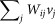, where, in general, vj could be a measure of the economic size of j. As explained below, here we take vj = 1 for all j. In order to take into account the impact of i on its indirect successors (that is, the nodes that can be reached from i and are at distance 2 or more), we introduce the following process. To each node we associate two state variables. hi is a continuous variable with hi [0, 1]. Instead, si is a discrete variable with 3 possible states, undistressed, distressed, inactive: si {U, D, I}. Denoting by Sf the set of nodes in distress at time 1, the initial conditions are: hi(1) = ψ i Sf; hi(1) = 0 i Sf and si(1) = D, i Sf; si(1) = U i Sf. The parameter ψ measures the initial level of distress: ψ [0, 1], with ψ = 1 meaning default. The dynamics is defined as follows,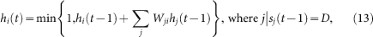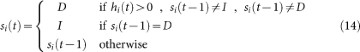for all i, where all variables hi are first updated in parallel, followed by un update in parallel of all variables si. After a finite number of steps T the dynamics stops and all the nodes in the network are either in state U or I. The intuition is that a nodes goes in distress when a predecessor just went in distress and so on recursively. The fraction of propagated distress is given by the impact matrix Wij. Because Wij ≤ 1 the longer the path from the node i initially in distress and node j, the smaller is the indirect impact on j. Notice that a node that goes in the D state, will move to the I state one step later. This means that if there is a cycle of length 2 the node will not be able to propagate impact to its successor more than once. This condition satisfies the requirement, mentioned earlier, of excluding the walks in which an edge is repeated.

The DebtRank of the set Sf is then defined as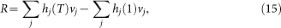i.e., R measures the distressed induced in the system, excluding the initial distress. If Sf is a single node the DebtRank measures the systemic impact of the node on the network. In this case, it is of interest to set ψ = 1 and to see the impact of a defaulting node. If Sf is a set of nodes it can be interesting to compute the impact of a small shock on the group. Indeed, while it is trivial that the default of a large group would cause the default of the whole network, it is not trivial to anticipate the effect of a little distress acting on the whole group.

The Group debt rank is a version of the DebtRank that computed the overall distress of the network shocking all the nodes with an initial shock ψ. Here we link ψ to the ratio of the house price index over disposal income in the following way.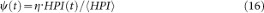Where 〈HPI〉 is the long term average of the house price index versus the household disposable income26 and η is an arbitrary constant that modulates the external shock. Data can be downloaded from the Federal housing finance agency (http://www.fhfa.gov)

As a final remark, we note that it would make sense to assign the market capitalization as proxy of size to each institution if we want to estimate its impact on the market. However, the market capitalization is strongly varying during the crisis and it can mask the other effects. In order to separate the network effects captured by the prices from the effects induced by the capitalization we used here a constant value for the node size.